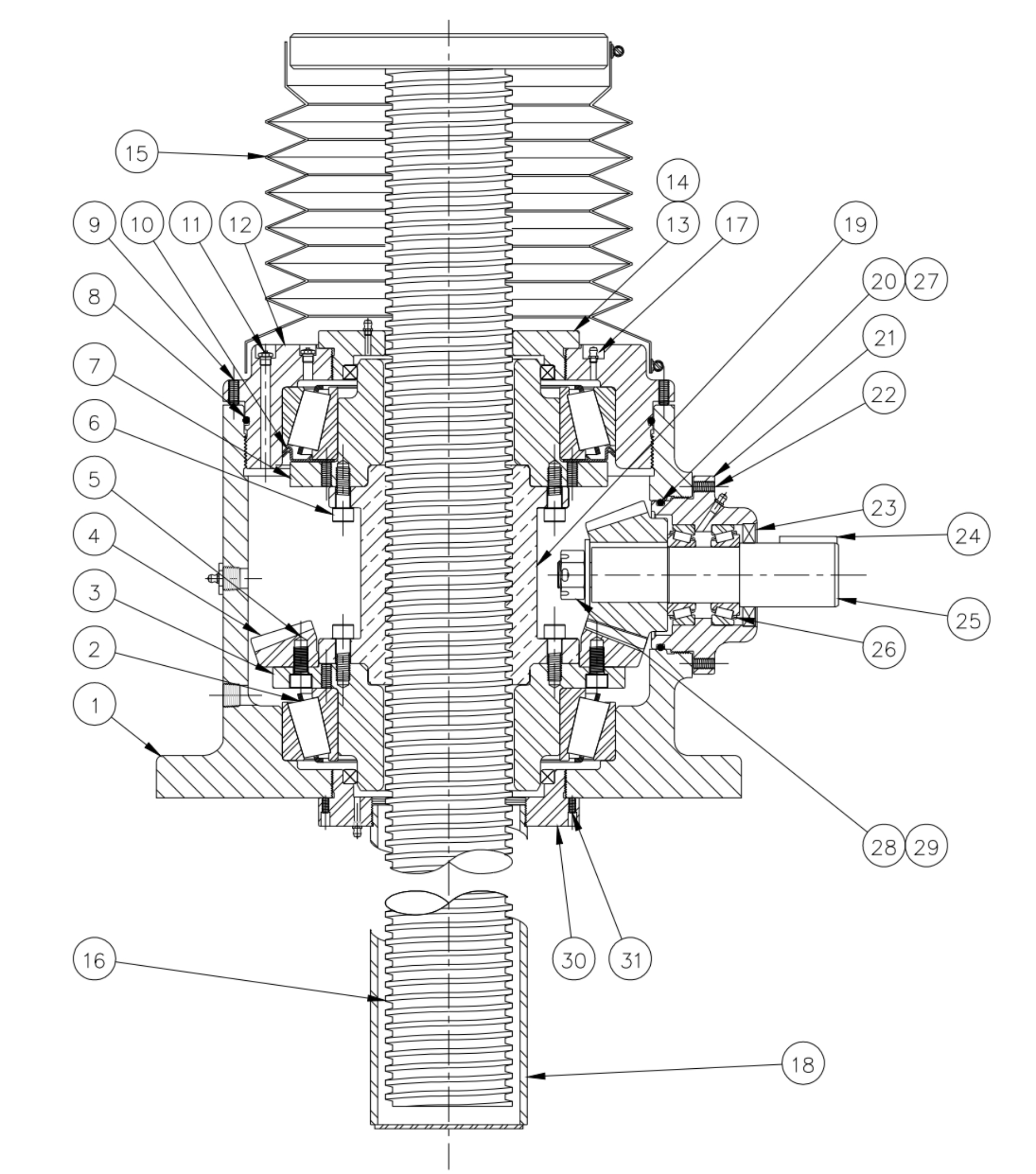# Bevel Gear Jack Application Information and Thermal Graphs# Bevel Gear Jack Application Information and Thermal Graphs

In many applications, Joyce Bevel Gear® jacks are more efficient and faster than wormgear driven jacks. To determine the suitability of a bevel gear jack for your application, use the steps below to calculate load, travel speed and duty cycle.

2. Determine velocity in feet / minute (fpm).
3. Determine duty cycle in terms of minutes operation / minutes resting (or time on / time off).
4. Calculate PV. PV = (load x velocity in fpm)/1000.
5. Calculate cooling time ( T ). T = Cooling time x time on/time off.
6. Plot the points for PV and T on the appropriate graph (below). If the point falls below the line, the application is satisfactory. If it is above the line, recalculate T for the next larger size jack. Each jack size has a different cooling time.
7. Calculate horsepower:RPM = Velocity in fpm x 12 x input turns per one-inch travel (from chart below). Horsepower = Pinion torque (from chart) x load x RPM.Note: PV = load x velocity (fpm)/1000 T = the maximum running time in minutes before a complete cooling time is required.

## Example

A 5000-pound load must be raised 30 inches in 15 seconds. The load remains in position for two minutes. It is then lowered and remains lowered for 30 seconds. The cycle begins again. Determine the appropriate bevel gear jacks and calculate horsepower required.